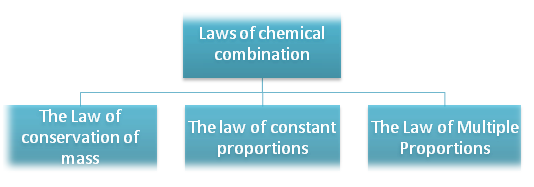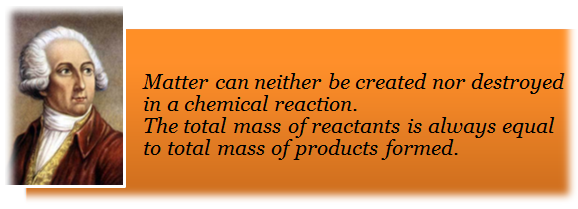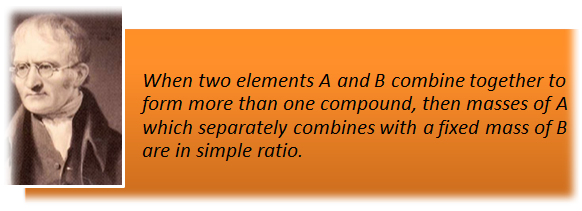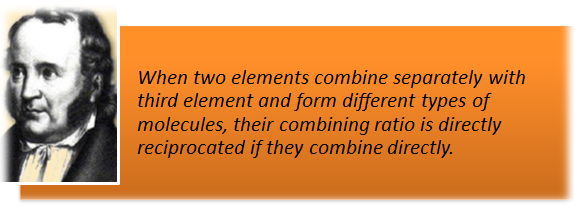×#### Thank you for registering.

One of our academic counsellors will contact you within 1 working day.

Click to Chat

1800-1023-196

+91-120-4616500

CART 0

• 0

MY CART (5)

Use Coupon: CART20 and get 20% off on all online Study Material

ITEM
DETAILS
MRP
DISCOUNT
FINAL PRICE
Total Price: Rs.

There are no items in this cart.
Continue Shopping```Laws of Chemical Combination

Table of Content

Law of Conservation of Mass

Example on Law of Conservation of Mass

Law of Constant Proportions

Examples on Law of Constant Proportions

Law of Multiple Proportion

Example on Law of Multiple Proportion

Law of Reciprocal Proportion

Example on Law of Reciprocal Proportion

Gay Lussac’s law of Combining Volumes

Example on Gay Lussac’s law of Combining Volumes

Related Resources

In order to understand the composition of the compounds, it is necessary to have a theory which accounts for both qualitative and quantitative observations during chemical changes. Observations of chemical reactions were most significant in the development of a satisfactory theory of the nature of matter. These observations of chemical reactions are summarized in certain statements known as laws of chemical combination.Law of Conservation of MassThis law was proposed by Antoine Lavoisier in 1744. According to this law, “Matter can neither be created nor destroyed in a chemical reaction”.

For any chemical change total mass of active reactants are always equal to the mass of the product formed.

It is a derivation of Dalton’s atomic theory ‘atoms neither created nor destroyed’.

Total masses of reactants = Total masses of products + Masses of unreacted reactants.

The exception to this law is nuclear reactions where mass is converted into energy. Here the Einstin’s Equation (E= mc2) is applicable.

Example on Law of Conservation of Mass

Question :

5.2 g of CaCO3 when heated produced 1.99 g of Carbon dioxide and the residue (CaO) left behind weighs 3.2g. Show that these results illustrate the law of conservation of mass.

Solution:

Weight of CaCO3  taken = 5.2 g
Total weight of the products (CaO +CO2)= 3.20 + 1.99 = 5.19 g

Difference between the wt. of the reactant and the total wt. of the products = 5.20 – 5.19 =0.01 g.

This small difference may be due to experimental error.

Thus law of conservation of mass holds good within experimental errors.

Law of Constant ProportionsThis law was proposed by Joseph Proust in 1799.

According to this law a chemical compound always contains same elements in definite proportion by mass and it does not depend on the source of compound.

This law can also be stated as “All pure samples of the same chemical compounds contain the same elements combined in the same proportion by mass”.

For exampleThe composition of CO2 obtained by different means always having same C:O ratio =Examples on Law of Constant Proportions

Question 1:

Ammonia  contains 82.65 % N2 and 17.65% H2. If the law of constant proportions is true, then the mass of zinc required to give 10 g Ammonia will be:

a. 8.265 g

b. 0.826 g

c. 82.65 g

d. 826.5 g

Solution:

The mass of zinc required to give 10 g ammonia will beHence (B) is correct.

_______________________________________________________

Question 2:

Irrespective of the source, pure sample of water always yields 88.89% mass of oxygen and 11.11% mass of hydrogen. This is explained by the law of:

a. conservation of mass

b. constant composition

c. multiple proportion

d. constant volume

Solution:

As in waterSo oxygen =Hydrogen =Both value always constant. Obey law of constant composition.

Hence (B) is correct.

________________________________________________________

Question 3:

6.488 g of lead combine directly with 1.002 g of oxygen to form lead peroxide. Lead peroxide is also produced by heating lead nitrate and it was found that the percentage of oxygen present in lead peroxide is 13.38 percent. Use these data to illustrate the law of constant composition.

Solution:

Step 1:

To calculate the percentage of oxygen in first experiment.

Weight of peroxide formed = 6.488 + 1. 002 = 7.490 g.

7. 490 g of lead peroxide contain 1.002 g of oxygen100 g of lead peroxide will contain oxygeni.e. oxygen present = 13.38%

Step 2:

To compare the percentage of oxygen in both the experiments.

Percentage of oxygen inin the first experiment = 13.38

Percentage of oxygen inin the second experiment = 13.38

Since the percentage composition of oxygen in both the samples ofis identical, the above data illustrate the law of constant composition.

___________________________________________________________________________

Question 4:

Copper oxide was prepared by the following methods:

a. In one case, 1.75 g of the metal were dissolved in nitric acid and igniting the residual copper nitrate yielded 2.19 g of copper oxide.

b. In the second case, 1.14 g of metal dissolved in nitric acid were precipitated as copper hydroxide by adding caustic alkali solution. The precipitated copper hydroxide after washing, drying and heating yielded 1.43 g of copper oxide.

c. In the third case, 1.45 g of copper when strongly heated in a current       of air yielded 1.83 g of copper oxide. Show that the given data illustrate the law of constant composition.

Solution:
Step 1:  In the first experiment.

2.19 g of copper oxide contained 1.75 g of Cu.100 g of copper oxide containedStep 2:  In the second experiment.

1.43 g of copper oxide contained 1.14 g of copper.100 g of copper oxide containedStep 3: In the third experiment.

1.83 g of copper oxide contained 1.46 g of copper100 g of copper oxide containedThus the percentage of copper in copper oxide derived from all the three experiments is nearly the same. Hence, the above data illustrate the law of constant composition.

Law of Multiple ProportionThis law was proposed by Jhon Dalton in 1804.

This law states that “When two elements combine to form two or more than two different compounds then the different masses of one element B which combine with fixed mass of the other element bear a simple ratio to one another.”

For example: Carbon forms two oxides in oxygen

Carbon monoxideCarbon oxideThe ratio of masses of oxygen in CO and CO2 for fixed mass of carbon (12) is 16 : 32 = 1: 2.

Example on Law of Multiple Proportion

Question 1:

The law of multiple proportions is illustrated by the pair of compounds:

a. sodium chloride and sodium bromide

b. water and heavy water

c. sulphur dioxide and sulphur trioxide

d. magnesium hydroxide and magnesium oxide

Solution:

In SO2 32 gram of suphur react with 32 gram of oxygen. Similarly for SO3 fixed mass of sulphur (32 gram) react with 48 gram of oxygen. The ratio of oxygen’s mass = 32:48 = 2:3\

Which support law of multiple proportions.

_______________________________________________________

Question 2:

Carbon is found to form two oxides, which contain 42.9% and 27.3% of carbon respectively. Show that these figures illustrate the law of multiple proportions.

Solution:
Step 1: To calculate the percentage composition of carbon and oxygen in each of the two oxides

First oxide

Second oxide

Carbon

42.9%

27.3%

Oxygen

57.1%

72.7%

Step 2:  To calculate the weights of carbon which combine with a fixed weight i.e., one part by weight of oxygen in each of the two oxides.

In the first oxide, 57.1 parts by weight of oxygen combine with carbon = 42.9 parts.1 part by weight of oxygen will combine with carbonIn the second oxide 72.7 parts by weight of oxygen combine with carbon = 27.3 parts.1 part by weight of oxygen will combine with carbonStep 3:  To compare the weights of carbon which combine with the same weight of oxygen in both the oxides- The ratio of the weights of carbon that combine with the same weight of oxygen (1 part) is 0.751: 0.376 or 2:1 Since this is a simple whole number ratio, so the above data illustrate the law of multiple proportions.

Law of Reciprocal ProportionThis law was proposed by “Richer” in 1794

This law states that ‘when two elements combine separately with third element and form different types of molecules, their combining ratio is directly reciprocated if they combine directly.”

The above law is the basis of law of equivalent masses, which will be described latter in details.

For example:ratio of masses of carbon and sulphur which combine with fixed mass (32 parts) of oxygen is

12:32 or 3:8                                  …(1)

Inratio of masses of carbon and sulphur is

12:64 or 3:16                     …(2)

The two ratios (1) and (2) are related to each other byor 2:1

ori.e. first ratio is integral multiple of second.

Example on Law of Reciprocal Proportion

Question 1 :

One part of an element A combines with two parts of B (another element). Six parts of element C combine with four parts of element B. If A and C combines together, the ratio of their masses will be governed by:

a. law of definite proportions

b. law of multiple proportions

c. law of reciprocal proportions

d. law of conservation of mass

Solution:from this when A combined with C the ratio is A/C = 1/3

Hence (C) is correct.

______________________________________________

Question 2:

Copper sulphide contains 66.6% Cu, copper oxide contains 79.9% copper and sulphur trioxide contains 40% Sulphur. Show that these data illustrates law of reciprocal proportions.

Solution:In copper sulphides

Cu : S mass ratio is 66.6 : 33.4

In sulphur trioxide

Oxygen : Sulphur (O:S) mass ratio is 60:40

Now in CuS

33.4 parts of sulphur combines with Cu = 66.6 parts

40.0 parts of sulphur combines with cuNow the ratio of masses of Cu and O which combines with same mass (40 parts) of sulphur separately is 79.8:60                …(1)

Cu : O ratio by mass in CuO is 79.9: 20.1         …(2)

Ratio 1: Ratio 2Which is simple whole number ratio, hence law of reciprocal proportion is proved.

Gay Lussac’s law of Combining VolumesAt given temperature and pressure the volumes of all gaseous reactants and products bear a simple whole number ratio to each other.

For examplei.e. one volume of hydrogen reacts with one volume of chlorine to form two volumes of HCl gas. i.e. the ratio by volume which gases bears is 1:1:2 which is a simple whole number ratio.

Example on Gay Lussac’s law of Combining Volumes

Question 1:

In the reaction,the ratio of volumes of nitrogen, hydrogen and ammonia is 1 : 3 : 2. These figures illustrate the law of:

a. constant proportions

b. Gay-Lussac

c. multiple proportions

d. reciprocal proportions

Solution:

The above ratio of 1 : 3 : 2 illustrates the Gay-Lussac law of combining volume.

Hence (B) is correct.

______________________________

Question 2:

How much volume of oxygen will be required for complete combustion of 40 ml of acetyleneand how much volume of carbondioxide will be formed? All volumes are measured at NTP.

Solution:40 ml40 ml                 100 ml                80 ml

So for complete combustion of 40 ml of acetylene, 100 ml of oxygen are required and 80 ml ofis formed.Question 1: Which of the following laws states that  “For any chemical change total mass of active reactants are always equal to the mass of the product formed.”

a.  Law of Conservation of Mass

b.  Law of Constant Composition

c.  Law of Multiple Proportions

d.  Gay Lussac’s law

Question 2: What would be the mass of water formed by reaction of two gram hydrogen with 16 gram oxygen?

a. 16 g

b. 32 g

c. 18 g

d. 20 g

Question 3: The statement ”Chemical compound always contains same elements in definite proportion by mass and it does not depend on the source of compound” belongs to

a.  Law of Conservation of Mass

b.  Law of Constant Composition

c.  Law of Multiple Proportions

d.  Gay Lussac’s law

Question 4:

Hydrogen combines with chlorine to form HCl. It also combines with sodium to form NaH. If sodium and chlorine also combine with each other, they will do so in the ratio of their masses as

a. 23 : 35.5

b. 35.5 : 23

c. 1 : 1

d. 23 : 1Q.1

Q.2

Q.3

Q.4

a

c

b

a

Related Resources

Look here for the syllabus of chemistry for  IIT JEE

You can also refer to Significant Figures and Scientific Notation Introduction

```### Course Features

• 731 Video Lectures
• Revision Notes
• Previous Year Papers
• Mind Map
• Study Planner
• NCERT Solutions
• Discussion Forum
• Test paper with Video Solution